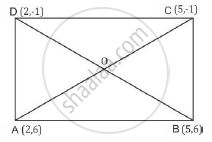# Prove that the diagonals of a rectangle ABCD, with vertices A(2, -1), B(5, -1), C(5, 6) and D(2, 6), are equal and bisect each other. - Mathematics

Prove that the diagonals of a rectangle ABCD, with vertices A(2, -1), B(5, -1), C(5, 6) and D(2, 6), are equal and bisect each other.

#### Solution 1

Solution:ΔADC and ΔBDC are right angled triangles with AD and BC as hypotaneus

AC^2=BA^2+BC^2

AC^2=(5-2)^2+(6+1)^2=9+49=58 sq.unit

BD^2=DC^2+CB^2

BD^2=(5-2)^2+(-1-6)^2=9+49=58 sq.unit

Hence, both the diagonals are equal in length.

#### Solution 2

The vertices of the rectangle ABCD are A(2, -1), B(5, -1), C(5, 6) and D(2, 6) Now,

"Coordinates of midpoint of" AC = ((2+5)/2 , (-1+6)/2) = (7/5 ,5/2)

"Coordinates of midpoint of " BD = ((5+2)/2 , (-1+6)/2)= (7/2,5/2)

Since, the midpoints of AC and BD coincide, therefore the diagonals of rectangle ABCD bisect each other.

Concept: Right-angled Triangles and Pythagoras Property
Is there an error in this question or solution?